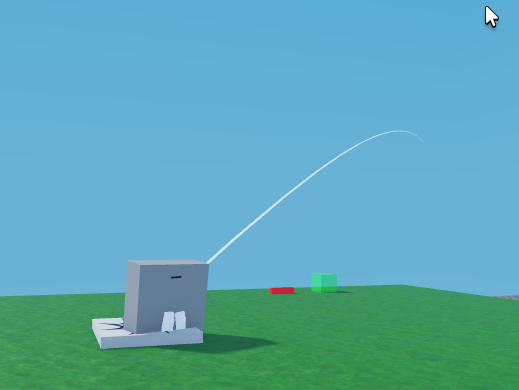# How to make beam always curve up

I want the beam to always curve up even if the attachments change orientation.

Attachment0 Parent = Handle – tool handleAttachment0 Parent = HumanoidRootPartHow can I always make it curve up?

Code:

``````local attach0 = Instance.new("Attachment")
local attach1 = Instance.new("Attachment")

AimBeam.Attachment0 = attach0
AimBeam.Attachment1 = attach1

attach0.Parent = HumanoidRootPart
attach1.Parent = Handle

local function beamProjectile(gravity, initialVelocity, initialPosition, duration)
local c = 0.5 * 0.5 * 0.5
local p3 = 0.5 * gravity * duration * duration + initialVelocity * duration + initialPosition
local p2 = p3 - (gravity * duration * duration + initialVelocity * duration) / 3
local p1 = (c * gravity * duration * duration + 0.5 * initialVelocity * duration + initialPosition - c * (initialPosition + p3)) / (3 * c) - p2

local curve0 = (p1 - initialPosition).Magnitude
local curve1 = (p2 - p3).Magnitude

local b = Vector3.new(0, 1, 0) -- Set the beam direction to always point upward
local r1 = (p1 - initialPosition).Unit
local u1 = b:Cross(r1).Unit
local r2 = (p2 - p3).Unit
local u2 = b:Cross(r2).Unit
b = r1:Cross(u1).Unit

local cf1 = CFrame.new(initialPosition.X, initialPosition.Y, initialPosition.Z, r1.X, u1.X, b.X, r1.Y, u1.Y, b.Y, r1.Z, u1.Z, b.Z)
local cf2 = CFrame.new(p3.X, p3.Y, p3.Z, r2.X, u2.X, b.X, r2.Y, u2.Y, b.Y, r2.Z, u2.Z, b.Z)

return curve0, -curve1, cf1, cf2
end

local function createAndSetBeam(gravity, initialVelocity, initialPosition, endTime)
local t1 = endTime or 1
local curve0, curve1, cf1, cf2 = beamProjectile(gravity, initialVelocity, initialPosition, t1)

AimBeam.CurveSize0 = curve0
AimBeam.CurveSize1 = curve1
AimBeam.Segments = 10 * math.round(t1 * 3)

attach0.CFrame = Handle.CFrame:Inverse() * cf1
attach1.CFrame = Handle.CFrame:Inverse() * cf2
end
``````

Never mind, I just fixed it! Forgot to change the Handle.CFrame:Inverse() to HumanoidRootPart.CFrame:Inverse().

This topic was automatically closed 14 days after the last reply. New replies are no longer allowed.